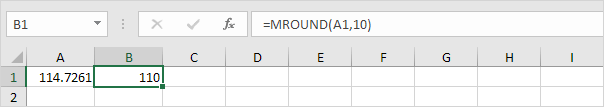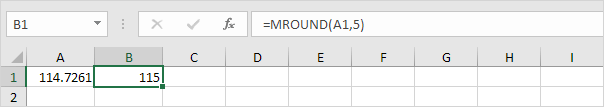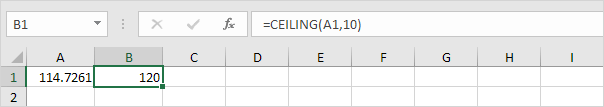# Nearest Multiple

This example illustrates three functions to round numbers to a multiple of x in Excel. The MROUND, CEILING and the FLOOR function.

### Mround

1. For example, round a number to the nearest multiple of 10.2. For example, round a number to the nearest multiple of 5.### Ceiling

The same as MROUND but rounds up.### Floor

The same as MROUND but rounds down.Go to Next Chapter: Formula Errors